Next: 3.1.2 The Drift-Diffusion Transport Up: 3.1 Sets of Partial Previous: 3.1 Sets of Partial

## 3.1.1 The Basic Semiconductor Equations

The basic equations are the Poisson equation and continuity equations for electrons and holes.

 div(. grad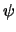) = q . (n - p - C) (3.2) div Jn = q .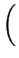R +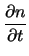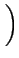(3.3) div Jp = - q .R +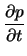(3.4)

The unknown quantities of this equation system are the electrostatic potentialand the electron and hole concentrations n and p, respectively. C denotes the net concentration of the ionized impurities,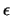is the dielectric permittivity of the semiconductor, and R is the net recombination rate which has to be modeled properly.

Tibor Grasser
1999-05-31